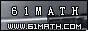Today is . WELCOME: huohai3983/44663  Home | Reg Login | All user | setHomePage | BookmarkHome | Math Test Online | Addition | Subtraction | Multiplication | Division | Time | Worksheet(JPG/PDF) | CAI | Flash Games |Recommendation articleArticle searchHome--maths--Third Grade--Adding Two-Digit Numbers with Paper and Pencil

Adding Two-Digit Numbers with Paper and Pencil

Adding Two-Digit Numbers with Paper and Pencil

How to add two two-digit numbers (for example 45 + 67) with paper and pencil.

• Write one number above the other so that the tens' place digits and ones' place digits are lined up. Draw a line under the bottom number.
```45
67
```
• Add the ones' place digits. (5 + 7 = 12). This sum is a two-digit number so place a one above the tens' place column and place the two below the line in the ones' place column.
```1
45
67
2
```
• Add the numbers in the tens' place column (1 + 4 + 6 = 11) and place the answer below the line and to the left of the ones' place sum.
``` 1
45
67
112```

#### Find the Sum of the two Numbers.

 +     =

2006-10-02 20:25:19
Viewed 2932 times CloseGreatmathsites